Wang, M., Wen, J., and Li, W. (2020). "Qualitative research: The impact of root orientation on coarse roots detection using ground-penetrating radar (GPR)," BioRes. 15(2), 2237-2257.

#### Abstract

The growth of coarse roots is complex, leading to intricate patterns of root systems in three dimensions. To detect and recognize coarse roots, ground-penetrating radar (GPR) was used. According to the GPR theory, a clear profile hyperbola is formed on the GPR radargrams when electromagnetic waves travel across two surfaces with different dielectric constants. First, the forward models (different root orientations) were built with simulation software (GprMax3.0) based on the finite-different time-domain method (FDTD). As the radar moved forward, the signal reflection curve was generated in different root orientations. An algorithm was proposed to obtain the coordinates of a single coarse root and analyze the influence of root direction on the hyperbola of coarse root through a symmetry curve and relative error (RE). Based on GPR datasets from the simulation experiment, the controlled experiment evaluated feasibility and effectiveness of the simulation experiment. To demonstrate the effect of the root orientation, the algorithm was applied to in situ recognition of the Summer Palace. The results showed that the localization of root orientation was relatively accurate. However, the proposed algorithm was unable to implement automatic detection, and the results still required human intervention. This research provides a solid basis for the biomass measurement, diameter estimation, and especially the three-dimensional reconstruction of ancient and famous trees.

Qualitative Research: The Impact of Root Orientation on Coarse Roots Detection Using Ground-Penetrating Radar (GPR)

Mingkai Wang,a,b Jian Wen,a,b,* and Wenbin Li a,b

The growth of coarse roots is complex, leading to intricate patterns of root systems in three dimensions. To detect and recognize coarse roots, ground-penetrating radar (GPR) was used. According to the GPR theory, a clear profile hyperbola is formed on the GPR radargrams when electromagnetic waves travel across two surfaces with different dielectric constants. First, the forward models (different root orientations) were built with simulation software (GprMax3.0) based on the finite-different time-domain method (FDTD). As the radar moved forward, the signal reflection curve was generated in different root orientations. An algorithm was proposed to obtain the coordinates of a single coarse root and analyze the influence of root direction on the hyperbola of coarse root through a symmetry curve and relative error (RE). Based on GPR datasets from the simulation experiment, the controlled experiment evaluated feasibility and effectiveness of the simulation experiment. To demonstrate the effect of the root orientation, the algorithm was applied to in situ recognition of the Summer Palace. The results showed that the localization of root orientation was relatively accurate. However, the proposed algorithm was unable to implement automatic detection, and the results still required human intervention. This research provides a solid basis for the biomass measurement, diameter estimation, and especially the three-dimensional reconstruction of ancient and famous trees.

Keywords: Coarse root systems; Root orientation; In situ recognition; Symmetry algorithm; Hough transform

Contact information: a: School of Technology, Beijing Forestry University, Beijing 100083, P. R. China; misslittlesheep@163.com; leewb@bjfu.edu.cn; b: Key Lab of State Forestry and Grassland Administration for Forestry Equipment and Automation, Beijing 100083, P. R. China;

* Corresponding author: wenjian@bjfu.edu.cn

INTRODUCTION

Coarse roots (> 2 mm in diameter), which are responsible for most root carbon storage, play a significant role in plant ecosystem function; they transfer water and nutrients (Dannoura et al. 2008). However, the study of coarse root systems is lacking because of the difficulty of underground observation and sampling. Traditional methods have many disadvantages in a large number of experiments such as considerable damage and operational complexity (Pransiska et al. 2016). Thus, non-destructive testing (NDT) methods are becoming popular for examining root systems (Hirano et al. 2009), due to their high efficiency and overall reliability of the information produced (Lv et al. 2018). Among these techniques, ground penetrating radar (GPR) is based on the scattering of electromagnetic waves radiating from a transmitting antenna. The radar signals form a reflection hyperbola at interfaces with different dielectric constants. Furthermore, the hyperbolic diffraction parameters (include two-way time delay, amplitude areas, pixels within the threshold range, mean pixel intensity, and reflector tally, etc.) are formed to locate the roots, evaluate the biomass, and draw the root configuration in the case of the difference of the electrical parameters between root and the surrounding soils (Hruska et al. 1999; Reubens et al. 2007).

Great achievements have been made in the field of root detection, such as root biomass detection and the automatic 3D reconstruction of roots. Hruška et al. (1999) first applied GPR for coarse root detection and mapping. The major interests of using GPR to detect coarse roots thus far include: 1) coarse roots mapping and 2) coarse roots biomass and diameter estimation. Barton and Montagu (2004) tested the ability of GPR with 500 MHz, 800 MHz, and 1 GHz antennas to detect tree roots and determine roots size by burying roots in a 32 m3 pit containing damp sand. The root diameters were predicted with a root mean squared error of 0.6 cm, which allows the detection and quantification of roots as small as 1 cm in diameter. Amato et al. (2008) tested the ability of two-dimensional (2-D) DC resistivity tomography to detect the spatial variability of roots and to quantify their biomass in a tree stand. This study provided a basis for developing quick nondestructive methods for detecting root distribution and quantifying root biomass. Zhu et al. (2014) established a feasible detection method to delineate the root distributions from the acquired 3D data by 3D GPR, and proposed some reasonable indexes for estimating root biomass (including the biomass of a single root and total biomass in the specific depth ranges) in field conditions. Liu et al. (2018) analyzed the relationship between the shape of the GPR signals and root orientation, and explored the equation of hyperbola signals reﬂected by roots. In sum, there are many factors (radar frequency, diameter of the root, dielectric constant) in the in situ identification and detection of roots. When these factors are controllable, the identification accuracy of root will be more accurate.

Previous work on the detection of coarse roots either focused on studying the effect of radar frequency on GPR radargrams or using 3D GPR to directly image coarse root systems. These studies indicate that in situ recognition of the root should be valued both in root detection and in 3-D architecture of coarse roots. The correct interpretation of GPR radargrams is the first step in root detection and mapping coarse roots. Because the spatial distribution of roots affects radar reflection imaging, the present study focused on the spatial distribution of roots and applying GPR for coarse root detection and quantification.

The emphasis of this paper is the detection of root orientation and in situ recognition of coarse roots. First, a mathematical model of GPR echo wave signals was developed. A forward model about coarse roots in different orientations was established, and a method of locating roots was proposed, which combined the hyperbolic symmetry algorithm with the Hough transform algorithm. Third, a controlled experiment was used to evaluate the feasibility and effectiveness of a simulation experiment based on GPR datasets. Finally, the proposed method was verified using simulation and in the ﬁeld experimental GPR data sets. The effect of root orientation on GPR hyperbolic is discussed.

EXPERIMENTAL

Materials

The GPR was purchased from TreeRadar Inc. (Silver Spring, MD, USA), which consists of a field data manager and a 900 MHz radar antenna. The experiment site was provided by the Summer Palace in Beijing.

Methods

Simulation model and GPR hyperbolic mathematical model

As shown in Fig. 1, the root (assuming it is completely straight for a finite length) was settled in a spatial rectangular coordinate system (the length and depth of the model are 0.24 m and 0.21 m, respectively) with one point in space as the original point. Both the root and the radar are in the positive coordinate system of space in order to display and analyze data more intuitively. The radar always moves along the same scan line, while the root rotates around its central point, which can construct roots in different orientations. The root orientation can been described by two parameters in the coordinate system: the horizontal orientation angle α (0° < α < 90°) and the vertical inclination angle β (0°<β<45°), as shown in Fig. 1a. The vertical inclination was defined as the angle of the projection of the root onto the y-z plane and z-axis. The azimuth angle was defined as the acute angle between the projection of the buried root onto the ground plane (x–z plane) and the orientation of the scanning line of the GPR (Liu et al. 2018).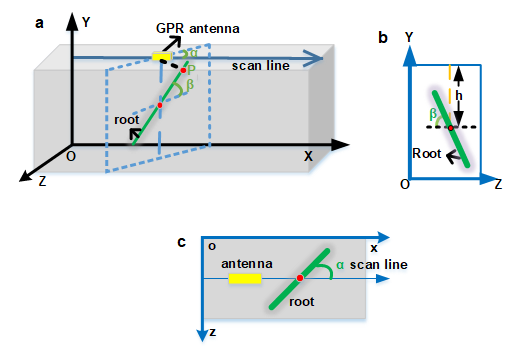Fig. 1. Illustration of the simulation experiment scenario in spatial rectangular. (a) The whole figure of model; (b) the left view of model; and (c) the top view of model coordinate system.

When the GPR moved along the scan line, there is always a point P on a single root that is closest to the radar. In other words, the point P is always the spot where the earliest arrival of electromagnetic waves from radar as well as the reflected signal back to a radar receiver, t represented as a the “round-trip” travel time for a path that runs from the transmitter to the object then back to the receiver. Therefore, the GPR radargrams contain information of about the root orientation, and other information.

According to the theory of GPR, only when the roots extend horizontally or vertically to the crosscutting line of the scan line will it contain valid information about the signals (e.g., root diameter or biomass). Therefore, the curve formula for the roots in this case (α = 90°, β = 0°) is needed. When the radar that sent and received electromagnetic waves in parallel is close to the ground or almost close to the ground, it is assumed that the propagation path in the soil that is homogeneous medium becomes relatively simple, then the electromagnetic wave only was reflected back to the receiving antenna after hitting the target. The projection point of a target on a radar detection line is x0, as shown in Fig. 2 (Lee and Mokji 2015). In addition, the t0 is a two-way travel time of x0, while z0 and h (shown in Fig. 1b) are equivalent to the depth of a single root center in the ground. The center of the single root is P when β = 0°. The β value affects the position of point P and the depth of the roots shown in the GPR radargrams. When GPR moves to xi or x0, the electromagnetic waves emitted by the transmitter can reach the surface of the root with minimum distance. Therefore, according to the triangle Pythagorean theory, the hyperbola is represented by the following equation.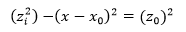(1)

where, z0 v·t0/2 and zv·ti/2, and zi is the straight-line distance between GPR and P in the general position. The v denotes the velocity of the wave in homogeneous medium. It can be expressed as equation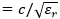, where c and r are, respectively, indicated as the speed of light and the relative permittivity of GPR detected soil. Further, the formula (1) becomes: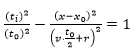(2)

Equation 2 conforms to the constraint equation of a hyperbola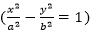. However, the diameters of coarse roots cannot be ignored. At the same time, the GPR wave propagation path will change, as shown in Fig. 2b. Equation 1 becomes transformed into Eq. 3. Adding zi and z0 in Eq. 3 creates Eq. 4.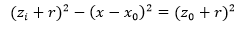(3)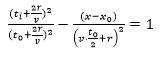(4)

From these expressions, the information of a single root in the ground will be included in the GPR radargrams as a hyperbola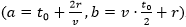.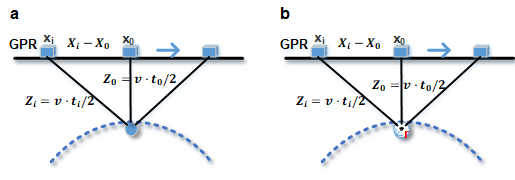Fig. 2. The formation process of hyperbola when the single root has a diameter and is ideal. (a) The ideal situation (r = 0); and (b) the case of the existence of diameter (r > 5 mm)

However, the shape of the coarse root is not always an ideal cylinder, due to the ecological environment of trees. Only when the shape of the oarse root nears a cylinder can the GPR reflection image fit with the constraint equation of the standard hyperbola. On the other hand, the hyperbola also contains the information of electromagnetic wave velocity and dielectric constant of medium besides the parameters of the coarse root. Next, the characteristics of electromagnetic wave velocity in root reflection hyperbola will be described.

Symmetry algorithm and Hough transform algorithm

In this section, as shown in Fig. 3, a new algorithm was proposed that combined the symmetry algorithm with the Hough transform algorithm. The most prominent features of hyperbolic signals are the symmetry properties between the vertices and the monotonic decrease on both sides of the vertices, as shown in Fig. 2. The symmetry curve (indicated as Eq. 5) of the hyperbola is the most important step in the symmetry algorithm, and it was obtained by similar methods as Eriksson and Papanikotopoulos (1997) and Prasad and Yegnanarayana (2004).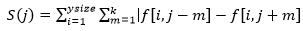(5)

In Eq. 5, ∈ [k+1, ysize-k]. In addition, the k is usually 0.2 or 1 of the effective caliber. The f [ij] is pixels of two-dimensional scan image obtained from GPR. The ysize and xsize are respectively the height and width of the image. The position corresponding to the minimum value of the symmetry curve S [j] is the horizontal position corresponding to the hyperbolic vertex in the image.

The procedure for extracting the vertex of the GPR hyperbola is as follows:

1) Preprocessing: remove the direct wave, background noise, median filtering, and mean filtering by using Matgpr (Tzanis 2013).

2) Edge extraction, ROI generation and edge binary in a ROI: canny edge detection and extract the region of interests through adaptive threshold method. Finally, the ROI is binary.

3) Extract symmetry curve: judge the extreme points of the symmetry curve of GPR hyperbola, the number of extremum points is the same as the number of roots when the symmetry curves are not intersect, or otherwise; we need analyze if the intersection of the symmetry curve matches the characteristics of the roots or not. The minimum point of symmetry curve was obtained using Matlab (MathWorks, Natick, MA, USA) algorithm: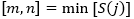(6)

where m is indicated as the scan number of hyperbola vertex, and the n is the minimum value of the symmetry curve.

4) Obtain the hyperbolic vertex delay: extract A-scan where the hyperbola vertex is located after extracting the horizontal position of hyperbola vertex. As shown in Fig. 4, the maximum amplitude point of the A-scan in the ROI is the time delay orientation coordinate (t) of the hyperbolic vertex.

To get the real depth of coarse roots, the electromagnetic wave velocity (v) of the medium is needed, as shown in Eq. 7.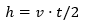(7)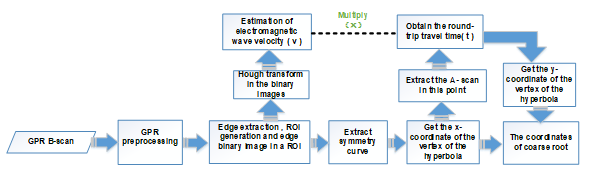Fig. 3. The algorithm process chart, showing the combination of the two algorithms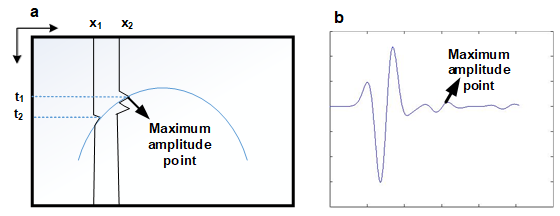Fig. 4. Illustration of the maximum amplitude point. (a) Maximum amplitude point in the B-scan; (b) maximum amplitude point in the A-scan

In previous work, the hyperbolic curve was shown to contain information about the electromagnetic wave velocity (v), because the v is inversely proportional to the relative permittivity (affects the configuration of the hyperbola). At present, the methods to estimate wave velocity from GPR data mainly include Hough transform (Al-Nuaimy et al. 2000; Golovko 2004), curve fitting (Osumi and Ueno 1985; Al-Nuaimy et al. 2001; Zhu et al. 2005), and waveform offset in the frequency domain (Xu and Miller 2001). However, the latter two methods require a large amount of computation. The estimation accuracy depends on the trial step size, so it is not convenient to give the variance of the estimation. The effectiveness of wave velocity estimation is evaluated by known target depth test method and imaging effect method. Hough transform uses the principle of dot-line duality that the points of the common line in the image correspond to the intersecting lines in the parameter space. Similarly, all curves intersecting at the same point in the parameter space have collinear points corresponding to them in the GPR B-scan. According to the above analysis, the GPR B-scan is firstly converted to the parameter space depicted in Eqs. 8 and 9. The original equation (the illustration in Fig. 5a) of the hyperbola is shown in Eq. 8,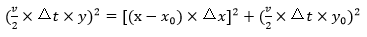(8)

where v represents the speed of electromagnetic wave propagation in soil, Δt represents the time interval or sampling rate of GPR data collection, and Δx represents the move spacing (we set to 5 mm in general) of GPR. The x0 is the x-coordinate of the vertex of the hyperbola, y0 is the y-coordinate of the hyperbola. Equation 9 gives the final transform: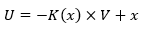(9)

where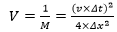(10)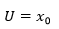(11)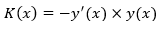(12)

In the above formulas, the hyperbolic equation has been converted to a linear parametric equation, as shown in Eqs. 9, 10, 11, and 12. Therefore after preprocessing and binary of hyperbola (shown in Fig. 5b), one y’(x) is obtained as long as a point is taken on the binary image. Combined with y(x), it is easier to get K(x). At the same time, countless points (-K(x)x) are obtained because the x is acquired. As a result, there is a line with a slope of –K(x) and an intercept of x that in the V-U domain of the Hough transform, as shown in the Fig. 5c. Thus, the electromagnetic velocity is calculated by Eq. 9 because v is contained in V, which is indicted as the intersection of many straight lines in the Hough coordinate system.

Subsequently, the h (the depth of coarse root or vertical coordinate of the coarse root position) was obtained in Eq. 7 based on the above conditions. The coordinate of single coarse root was known in the rectangular coordinate system (Fig. 1) as the x (the horizontal coordinate of the coarse root position) can be transformed from m (shown in Eqs. 6 and 15).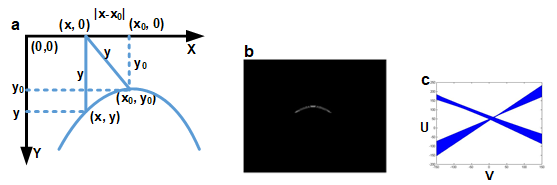Fig. 5. (a) Mathematical form of the Hough Transformation of a hyperbola; (b) preprocessed in the original B-scan and edge extraction, ROI generation and edge binary in a ROI; (c) Hough Transform in the V-U domain

Simulation experiment, controlled experiment, and field experiment

In general, field experiments validate the feasibility of the algorithms that are used in the simulation experiments. Moreover, a simulation experiment is crucial in the whole inversion. The above models (shown in Fig. 1) were built with GprMax 3.0 software (University of Edinburgh, Dr. Antonis Giannopoulos, UK) based on Maxwell equations and the ﬁnite-different-time-domain theory (Giannopoulos 2005; Li et al. 2012). The root was 2 cm, and the waveform was a Ricker with an amplitude current of 1 A. For the convenience of radar detection, root depth was set to 10 cm. The scan number of GPR was set to 60. The time window was set to 3 ns, and the increment of each step of the antenna was 0.002 m. The dielectric constant permittivity of the soil and the root were 5 and 10, respectively. The dx, dy, and dz (PML boundary condition that can eliminate the noise caused by model boundary) were set to 0.002 m (Feng and Dai 2011). According to previous research findings, the center frequency of the radar was set as 900 MHZ, because this radar resolution is more suitable for shallow root detection (Hirano et al. 2009).

Table 1. Summary of Field Experiment in Summer Palace with Willow, Pine, and Cypress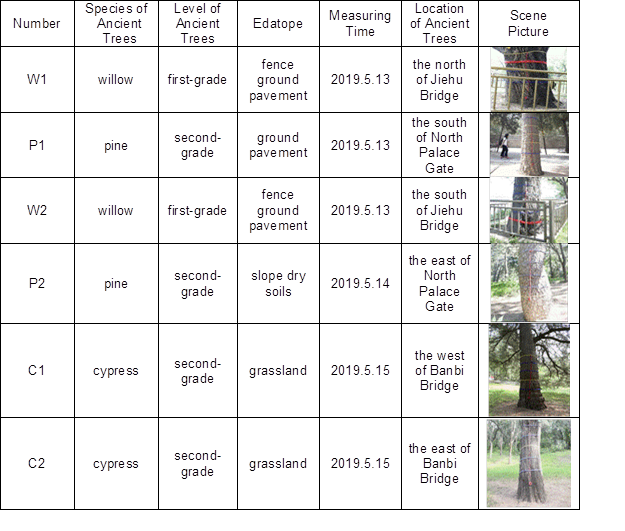In the simulation experiment, the root center was in the center of the model, and the root orientation was changed as it rotated around its center in a clockwise orientation. Based on the above factors, radar reflection images were obtained in different orientations of a single coarse root. These images can really well simulate the scene of different root orientations. Additionally, the simulated images were visualized by use of the imaging command in Matlab and underwent imagery preprocessing to enhance the image quality.

To verify the validity of simulaiton experiments, the controlled experiments in different root directions were carried out in the Gold Plant (40°29″N, 116°20′27″ E). The region has a temperate continental monsoon climate with four distinct seasons. In the controlled experiment, grapevine was selected to simulate the detection of coarse roots. The surface of the study area is mainly fixed dune, and the soil type is mainly fine sand (Fig. 6a). The permittivity of natural fine sand is close to that of soil, and the physical properties are uniform. No rainfall occurred during the experiment, so the soil moisture content was stable and suitable for GPR detection.

In the controlled experiment, four roots with an average diameter of 2 cm were placed in a trench 2 m long and 1 m wide. The burial depth was 10 cm. The average water content of root was more than 30% because roots having high volumetric water content were easily detected (Guo et al. 2013). The soil dielectric constant was set to 5. To simulate roots with different vertical inclinations (Fig. 6a), the roots were obliquely inserted into the soil. Meanwhile, the radar scaned each root at different scanning angle (Fig. 6b). Finally, radar reflection images with different root directions were obtained in GPR radargrams.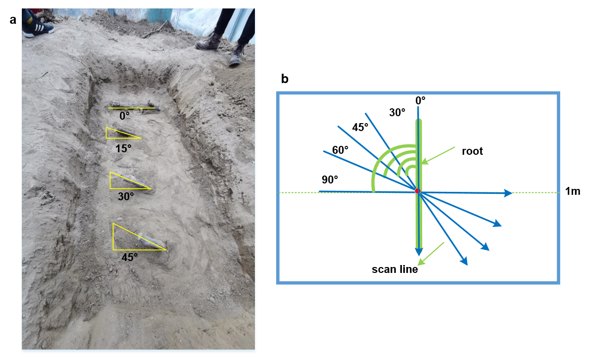Fig. 6. (a) Field diagram of roots with different vertical inclinations ( = 0, 15, 30, 45); (b) the top view of schematic scene in different scanning angles of radar ( = 90, 60, 45, 30, 0)

The ﬁeld experiment was performed on the grounds of the Summer Palace (39°59′29″N, 116°16′19″E). The Summer Palace, which is located in Haidian district of Beijing, has a temperate monsoon climate. The main vegetation in Park vegetation are cypress, pine, and willow; their roots are all coarse.

Table 1 shows the summary of the field experiment that contains test records for six trees. Two regions were selected for analysis: (1) the north of Banbi Bridge, and (2) the south of North Palace Gate (Fig. 7).

In general, the simulation experiment and controlled experiment can draw some correct conclusions. Distinguished by means of experiments under ideal conditions, there were many variables in the field. The pine and cypress trees were the main detection objects due to their difference in root growth. In the field, the orientation of the roots is uncertain because of the complexity of actual root growth. Nonetheless, the simulation experiment and controlled experiment can be used as prior knowledge to provide a basis for field experiments.